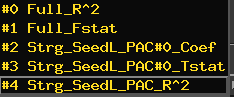# Beta-series Seed2WB correlation maps

Hi Folks,

Loosely following Andrew Jahn’s BetaSeries tutorial https://www.andysbrainblog.com/andysbrainblog/2014/05/creating-beta-series-correlation-maps.html and he has 3dfim+ for the seed/ideal to whole brain correlation step. However help file for 3dfim+ appears to say its old and not being maintained. Is 3dDeconvolve now the preferred approach these days?

If so:

A. What is proper polort # and other basic parameters to use?
e.g. - stim_times_1 for seed?
- 6 motion variables + censor file (even necessary if was part of original beta-series estimation step?)
- R out, f out, t out
- basis_normall 1
- bucket, etc…?

B. What is the proper Coef and R^2 subbriks outputs to use in the following square root step ‘ispositive(b)*sqrt(a)-isnegative(b)*sqrt(a)’ equation, the FULL_model, or the Stim_file_1_SEED?

Help, as always, is very much appreciated,

~Dane

help file for 3dfim+ appears to say its old and not being maintained. Is 3dDeconvolve now the preferred approach these days?

Even though 3dfim+ will not be updated, it can still be used as long as you adopt the same or similar model formulations. In fact, you can directly obtain the output for correlation values from 3dfim+ without conversion like 3dDeconvolve.

What is proper polort # and other basic parameters to use?

The order of polynomial fit is purely empirical. If I remember it correctly, a rule of thumb is one order per 150 seconds of data. For Beta Series analysis, probably easier to use the option -stim_times_IM.

What is the proper Coef and R^2 subbriks outputs to use in the following square root step
‘ispositive(b)*sqrt(a)-isnegative(b)*sqrt(a)’ equation, the FULL_model, or the Stim_file_1_SEED?

The ‘a’ part is the regression coefficient and ‘b’ is R^2.

Very helpful Gang. I hope you can bear with a few clarification questions ~Dane

help file for 3dfim+ appears to say its old and not being maintained. Is 3dDeconvolve now the preferred approach these days?

Even though 3dfim+ will not be updated, it can still be used as long as you adopt the same or similar model formulations. In fact, you can directly obtain the output for correlation values from 3dfim+ without conversion like 3dDeconvolve.

>>> What exactly do you mean by “similar model formulations”? Do you mean using same polort# and Pearson’s vs Spearman’s correlation, or something else? e.g. perhaps “modeling” like stim_time_IM vs AM, and block vs. tent? If so, how would you specify it in 3dfim+?

What is proper polort # and other basic parameters to use?

The order of polynomial fit is purely empirical. If I remember it correctly, a rule of thumb is one order per 150 seconds of data. For Beta Series analysis, probably easier to use the option -stim_times_IM.

[u][b]>>> From a theoretical standpoint, is use of polort deconvolution even necessary if it was already applied at previous step to create the Betaseries estimations used in this subsequent correlation step? If not, would one use polort 0 or 1 to not apply it?

In regards to stim_times_IM, do you mean using this in the second 3ddeconvolve when labeling the seed signal for correlation to wholebrain?[/b][/u]

What is the proper Coef and R^2 subbriks outputs to use in the following square root step
‘ispositive(b)*sqrt(a)-isnegative(b)*sqrt(a)’ equation, the FULL_model, or the Stim_file_1_SEED?

The ‘a’ part is the regression coefficient and ‘b’ is R^2.

>>> Apologies I think this question was confusing. In the bucket file output there are two groups of subbrik outputs: the “Full model” (#0 & #1) and “the seed” as specified in the stim_time1 (#2 thru #4). See image attached from the GUI. Both can have coef and R^2. Just not sure which represents the desired correlation.What exactly do you mean by “similar model formulations”? Do you mean using same polort# and
Pearson’s vs Spearman’s correlation, or something else? e.g. perhaps “modeling” like stim_time_IM
vs AM, and block vs. tent? If so, how would you specify it in 3dfim+?

For task-related data, better switch to 3dDeconvolve. It’s inconvenient to deal with task-related regressors with 3dfim+. However, it could be more convenient using 3dfim+ for beta-series correlation.

From a theoretical standpoint, is use of polort deconvolution even necessary if it was already applied at previous step to create the Betaseries estimations used in this subsequent correlation step? If not, would one use polort 0 or 1 to not apply it?

To compute correlation, it makes sense to remove the mean with -polort 0

In regards to stim_times_IM, do you mean using this in the second 3ddeconvolve when labeling the seed signal for correlation to wholebrain?

I was referring to obtaining the trial-level betas that you would later use for beta-series correlation analysis.

In the bucket file output there are two groups of subbrik outputs: the “Full model” (#0 & #1) and
“the seed” as specified in the stim_time1 (#2 thru #4). See image attached from the GUI. Both
can have coef and R^2. Just not sure which represents the desired correlation.

Only sub-bricks 2 and 4 in your image are relevant to your beta-series correlation analysis.